5.4.0

# Fixed Port Range IP Pools algorithm

IP pools are a useful tool in NATing where the basic principles are fairly straightforward and the more basic options are used with great success. However, this SysAdmin Note is going to concentrate on one of the lesser used IP Pool types – Fixed Port Range. To focus on something even more low level, we’re going to look at how the IP addresses and ports are selected.

In a Fixed Port Range type of IP pool there is nothing to force the internal and external ranges to be the same size so there isn’t an obvious way to predict what the external IP address is going to be based on the internal or source address. There is no one-to-one relationship between the addresses.

Here’s an example scenario for when you might want to use Fixed Port Range IP pool:

• Internal IP subnet of computers using an IP pool: 172.16.4.1 - 172.16.7.255. This means there are 1022 usable IP addresses.
• The company has what used to be called a C-class address which is 254 usable addresses on the Internet. Of these, 64 have been set aside for the IP pool.

While there isn’t any obvious method of prediction, in some situations there can be a requirement to be able to predict the relationship between the source IP address and the one that it is given from the IP pool. This can occur in situations, that for security reasons, the public IP address of a session needs to be known because communications between sites are limited to specific IP addresses.

## Determining the IP address from the pool

I apologize for those that hoped they wouldn’t have to deal with math and formulas after leaving high school or college but let’s face it, computers are all about numbers. The following is the logic used to determine the IP address.

### Defining the variables

The first step in having the algorithm make sense is to define the variables we will be using:

Variable Name

Description

src_start

Starting IP address of the IP pool’s source IPs

src_end

End or last IP address of the IP pool’s source IPs

start

Starting IP of the IP pool’s translated IP addresses

end

End or last IP of the IP pool’s translated IP addresses

src_ip

Incoming IP address from the source device. This will fall in the range [src_start, src_end])

### The equations

Now that we have defined the variables, we can start to place them in the algorithm. In order to make the algorithm more manageable and understandable, it is broken into 2 parts.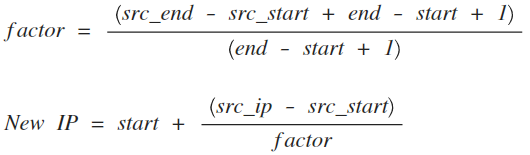Note: You should be aware that floating point calculations are not used so the result from the factor calculation will be an integer. The value is truncated, not rounded, so 36/10=3, not 4, as would be the case if rounded to the nearest integer.

### Setting values for the example

Because spanning multiple subnets make the math trickier we are going to use a simpler example of a Fixed Port Range IP pool.

Variable

Value

Source (internal) range

192.168.1.100 – 192.168.1.200

External range

10.1.1.50 – 10.1.1.80

160

### First equation – determining the factor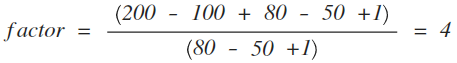### Second equation – determining the IP address from the pool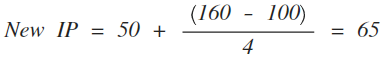If a computer with the IP address 192.168.1.160 initiates a session through a policy using the example IP pool, 10.1.1.65 will be assigned as the NATed address.

These calculations get more complicated when you overlap subnets, but this example should give the basic principles behind the process for assigning IP addresses.

## TCP ports

The TCP port being use in a session could also be used to help determine which computer is the originating source of the session, if it could be narrowed down far enough. This is more difficult than working with the IP addresses. The problem is that determining which ports are assigned can have less to do with a unique computer identity than the order in which he sessions were initiated.

There can be some narrowing down from the total of possibilities, but normally the scope of probable TCP ports is a larger range of numbers than the scope of IP addresses.

### Defining the variables

Just like we did when determining the IP addresses, we’ll start by defining some variables and values.

Variable name

Description

snat_port_begin

The beginning of the range of NATed ports

snat_port_end

The end of the range of NATed ports

port_share

The range size that the total number of available ports will be divided into in order to distribute the ports among the sessions

first_port_choice

The number the port that the system will try to use first for the session

• src_ip
• src_start
• factor

These variable were all defined in the previous algorithm

### Setting values for the example

Now that we know what all of the variables mean, let’s give some values to a few in order to begin the calculations.

Variable

Value

snat_port_begin 5117
snat_port_end 65533

These values are the default ones in the system. With an entire port range of possibilities from 5117 (snat_port_begin) to 65533 (snat_port_end), It makes for a large number of possibilities. It does get narrowed down a little by using the factor equation (see the section for calculating the IP addresses) to divide the range into shares for the sessions to use.

### The equations

This equation is used to divide the total number of available ports into port shares that are of equal size for the sessions to be divided between.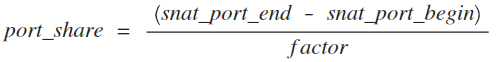To determine the first choice of ports for a session, second equation is used.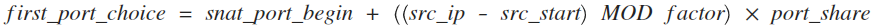This equation make use of the modulus function, of as shown in the equation, MOD. The use of MOD makes this equation a little more interesting because we are multiplying by the remainder, instead of the resulting value from that portion of the equation. This has the effect of distributing the ports for session not like a continuous stream but more like dealing out cards from a deck to players. Session from IP address x goes to port share 1. Session from IP address x+1 goes to port share 2 and so on until we run out of shares that we start over from the beginning.

Using the information from the previous example, walking the calculations through step by step looks like this:

### First equation – determining the port share

We know the factor is 4, so determining the port_share is straightforward.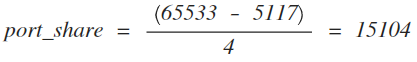### Second equation – determining the first port choice for the session

Before running the whole second equation let’s solve the MOD portion.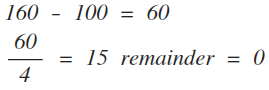It happens in this case that 4 went into 60 cleanly with no remainder, making the value 0 and forcing us to multiply by 0, which nobody likes, but it will still work in the final equation. If the src_ip was 162, the remainder would have ended up being 2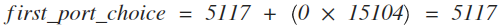To add a little ambiguity, the value produced by this equation does not guarantee that it will be the port used. Ports are assigned on a first come, first served basis. If the first_choice_port is being used by another session already, then the FortiGate will use generic logic going up the port list incrementally, to search for the next available port.

This methodology in determining the port used for a session tries to strike the balance between assigning specific port numbers and making sure that every session can be assigned a port. Because some computers will generate a lot more sessions than others a certain amount of flexibility has to be built in. With that flexibility comes unpredictability.

If the options for the port were limited to the scope of the port_share, one could expect the options for the ports assigned to sessions from the IP address in the example to be anywhere from 5117 to 20220. 20220 being the number before the first_port_choice in the next port share. If the remainder for the MOD portion of the equation been 1 instead of 0, the first_port_choice value would have been 20221. However, because the system keeps going up the port list until it finds a free one, if for some reason all of the ports in the first range are already in use, the system will keep going through the list until it finds a free port, even if it is a number above 20221.

The algorithm for assigning ports works very will for making sure that whenever possible, every attempted session gets a port for the purposes of NATing. The drawback is that it, while it gives a probable port range, it doesn’t guarantee the sessions will be within the range. This means that reversing the algorithm to determine the source computer for the purposes of forensic analysis will not give a definite conclusion.

# Fixed Port Range IP Pools algorithm

IP pools are a useful tool in NATing where the basic principles are fairly straightforward and the more basic options are used with great success. However, this SysAdmin Note is going to concentrate on one of the lesser used IP Pool types – Fixed Port Range. To focus on something even more low level, we’re going to look at how the IP addresses and ports are selected.

In a Fixed Port Range type of IP pool there is nothing to force the internal and external ranges to be the same size so there isn’t an obvious way to predict what the external IP address is going to be based on the internal or source address. There is no one-to-one relationship between the addresses.

Here’s an example scenario for when you might want to use Fixed Port Range IP pool:

• Internal IP subnet of computers using an IP pool: 172.16.4.1 - 172.16.7.255. This means there are 1022 usable IP addresses.
• The company has what used to be called a C-class address which is 254 usable addresses on the Internet. Of these, 64 have been set aside for the IP pool.

While there isn’t any obvious method of prediction, in some situations there can be a requirement to be able to predict the relationship between the source IP address and the one that it is given from the IP pool. This can occur in situations, that for security reasons, the public IP address of a session needs to be known because communications between sites are limited to specific IP addresses.

## Determining the IP address from the pool

I apologize for those that hoped they wouldn’t have to deal with math and formulas after leaving high school or college but let’s face it, computers are all about numbers. The following is the logic used to determine the IP address.

### Defining the variables

The first step in having the algorithm make sense is to define the variables we will be using:

Variable Name

Description

src_start

Starting IP address of the IP pool’s source IPs

src_end

End or last IP address of the IP pool’s source IPs

start

Starting IP of the IP pool’s translated IP addresses

end

End or last IP of the IP pool’s translated IP addresses

src_ip

Incoming IP address from the source device. This will fall in the range [src_start, src_end])

### The equations

Now that we have defined the variables, we can start to place them in the algorithm. In order to make the algorithm more manageable and understandable, it is broken into 2 parts.Note: You should be aware that floating point calculations are not used so the result from the factor calculation will be an integer. The value is truncated, not rounded, so 36/10=3, not 4, as would be the case if rounded to the nearest integer.

### Setting values for the example

Because spanning multiple subnets make the math trickier we are going to use a simpler example of a Fixed Port Range IP pool.

Variable

Value

Source (internal) range

192.168.1.100 – 192.168.1.200

External range

10.1.1.50 – 10.1.1.80

160

### First equation – determining the factor### Second equation – determining the IP address from the poolIf a computer with the IP address 192.168.1.160 initiates a session through a policy using the example IP pool, 10.1.1.65 will be assigned as the NATed address.

These calculations get more complicated when you overlap subnets, but this example should give the basic principles behind the process for assigning IP addresses.

## TCP ports

The TCP port being use in a session could also be used to help determine which computer is the originating source of the session, if it could be narrowed down far enough. This is more difficult than working with the IP addresses. The problem is that determining which ports are assigned can have less to do with a unique computer identity than the order in which he sessions were initiated.

There can be some narrowing down from the total of possibilities, but normally the scope of probable TCP ports is a larger range of numbers than the scope of IP addresses.

### Defining the variables

Just like we did when determining the IP addresses, we’ll start by defining some variables and values.

Variable name

Description

snat_port_begin

The beginning of the range of NATed ports

snat_port_end

The end of the range of NATed ports

port_share

The range size that the total number of available ports will be divided into in order to distribute the ports among the sessions

first_port_choice

The number the port that the system will try to use first for the session

• src_ip
• src_start
• factor

These variable were all defined in the previous algorithm

### Setting values for the example

Now that we know what all of the variables mean, let’s give some values to a few in order to begin the calculations.

Variable

Value

snat_port_begin 5117
snat_port_end 65533

These values are the default ones in the system. With an entire port range of possibilities from 5117 (snat_port_begin) to 65533 (snat_port_end), It makes for a large number of possibilities. It does get narrowed down a little by using the factor equation (see the section for calculating the IP addresses) to divide the range into shares for the sessions to use.

### The equations

This equation is used to divide the total number of available ports into port shares that are of equal size for the sessions to be divided between.To determine the first choice of ports for a session, second equation is used.This equation make use of the modulus function, of as shown in the equation, MOD. The use of MOD makes this equation a little more interesting because we are multiplying by the remainder, instead of the resulting value from that portion of the equation. This has the effect of distributing the ports for session not like a continuous stream but more like dealing out cards from a deck to players. Session from IP address x goes to port share 1. Session from IP address x+1 goes to port share 2 and so on until we run out of shares that we start over from the beginning.

Using the information from the previous example, walking the calculations through step by step looks like this:

### First equation – determining the port share

We know the factor is 4, so determining the port_share is straightforward.### Second equation – determining the first port choice for the session

Before running the whole second equation let’s solve the MOD portion.It happens in this case that 4 went into 60 cleanly with no remainder, making the value 0 and forcing us to multiply by 0, which nobody likes, but it will still work in the final equation. If the src_ip was 162, the remainder would have ended up being 2To add a little ambiguity, the value produced by this equation does not guarantee that it will be the port used. Ports are assigned on a first come, first served basis. If the first_choice_port is being used by another session already, then the FortiGate will use generic logic going up the port list incrementally, to search for the next available port.

This methodology in determining the port used for a session tries to strike the balance between assigning specific port numbers and making sure that every session can be assigned a port. Because some computers will generate a lot more sessions than others a certain amount of flexibility has to be built in. With that flexibility comes unpredictability.

If the options for the port were limited to the scope of the port_share, one could expect the options for the ports assigned to sessions from the IP address in the example to be anywhere from 5117 to 20220. 20220 being the number before the first_port_choice in the next port share. If the remainder for the MOD portion of the equation been 1 instead of 0, the first_port_choice value would have been 20221. However, because the system keeps going up the port list until it finds a free one, if for some reason all of the ports in the first range are already in use, the system will keep going through the list until it finds a free port, even if it is a number above 20221.

The algorithm for assigning ports works very will for making sure that whenever possible, every attempted session gets a port for the purposes of NATing. The drawback is that it, while it gives a probable port range, it doesn’t guarantee the sessions will be within the range. This means that reversing the algorithm to determine the source computer for the purposes of forensic analysis will not give a definite conclusion.